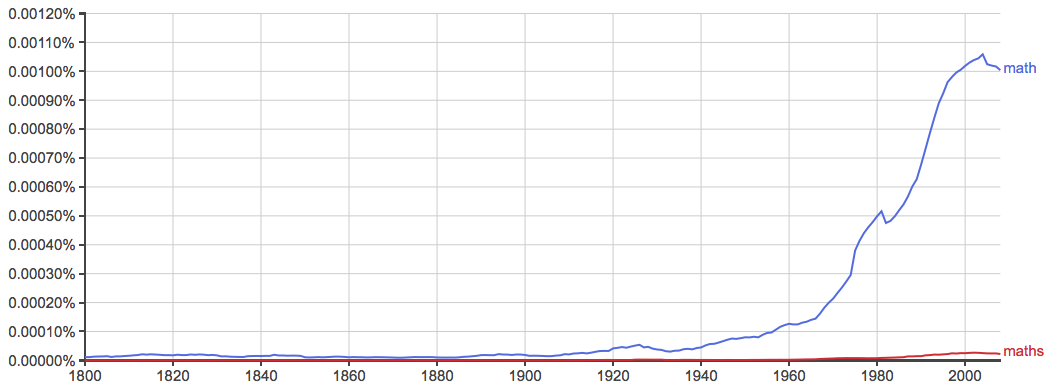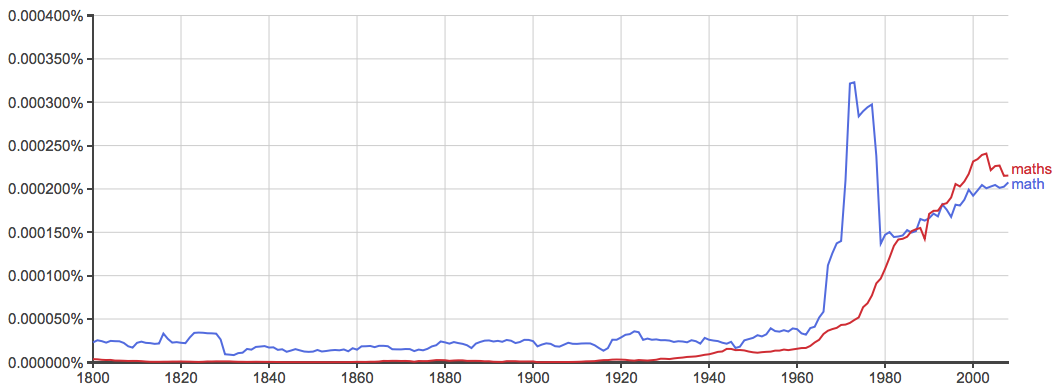# Math or Maths – What’s the Difference?

By any name, writers as a group have encountered no enemy quite so intractable as the enemy of mathematics. Writers don’t understand numbers. We don’t trust them. Nonetheless, mathematics is part of life, and we must learn to accept the things we can’t change.

As if the numbers themselves weren’t confusing enough, there are two ways to shorten mathematics—as math and as maths. Luckily, we writers like words, and we can handle simple dilemmas such as this one.

Both of these terms are correct. If you don’t know when to choose math or maths, you aren’t alone. Continue reading to discover the proper usage cases for each of these words.

## What is the Difference Between Math and Maths?

In this article, I will compare math vs. maths. I will use each of these spellings in at least one example sentence, so you can see them in context.

Plus, I will show you a useful mnemonic that will help you next time you need to choose either maths or math.

## When to Use MathWhat does math mean? Math is a shortened form of the word mathematics, which is the study of number, quantity, and space. Math is a crucial element of such pursuits as physics, engineering, accounting, statistical analysis, and basic personal finance. It is also a field of study in itself.

Here are a few examples of math used in sentences.

• I have math homework to do, but I would rather do something else.
• Early intervention for math should target either procedural fluency or conceptual understanding.
• “We throw away a banana for every buck we take, so no one finds out,” Maebe said, to which T-Bone replied, “I think you should do that math again.”
• But reading and math scores were essentially flat on the most recent Nation’s Report Card, a measure of student achievement. –The Wall Street Journal

Math is the predominant form of this word in American English.As you can see in the above chart, math is the clear winner in American English.

## When to Use MathsWhat does maths mean? Maths is another form of the same word. Where math is prevalent in American English, maths is much more common in British English.

It is common to see maths is British publications.

Osborne said schools in the north should aim to raise attainment at 16 in English and maths to be at least the national average, and that he wanted the north to be regarded as a leading European region in digital skills at 16. –The Guardian

Interestingly, the British have preferred math for most of the 19th and 20th centuries, culminating in a prominent spike in usage around 1970, before maths overtook it.## Trick to Remember the Difference

Math and maths are the standard forms of this word in American and British English, respectively.

Luckily, there is a very easy way to remember maths vs. math. Maths ends in the letter S, just like the river Thames in England. If you can link this bit of British geography to the British word maths, you should have no trouble remembering the usage cases for these variants.

## Summary

Is it math or maths? Both maths and math are shortened forms of the word mathematics, which is the study of number, quantity, and space.

• Math is the American variant.
• Maths is the British variant.

Since maths and Thames both end with an -s, you should have little trouble remembering when to use maths.

Math is difficult, but even if you don’t understand mathematics, you can be sure you’re using the correct word when you write about it.

Contents